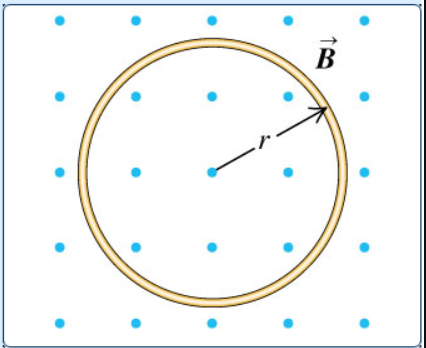# Problem: A circular loop of wire with radius r = 0.0480 m and resistance R = 0.160 Ω is in a region of spatially uniform magnetic field, as shown in the following figure. The magnetic field is directed out of the plane of the figure. The magnetic field has an initial value of  7.63 T and is decreasing at a rate of –0.602 T/s. What is the rate at which electrical energy is being dissipated by the resistance of the loop?Express your answer with the appropriate units.P = ?

###### FREE Expert Solution

In this problem, we are dealing with induced emf due to a changing magnetic flux.

Induced emf:

$\overline{){\mathbf{\epsilon }}{\mathbf{=}}{\mathbf{-}}{\mathbit{N}}\frac{\mathbf{d}{\mathbf{\varphi }}_{\mathbf{B}}}{\mathbf{d}\mathbf{t}}}$

Magnetic flux:

$\overline{){\mathbf{\varphi }}{\mathbf{=}}{\mathbf{B}}{\mathbf{A}}{\mathbf{c}}{\mathbf{o}}{\mathbf{s}}{\mathbf{\theta }}}$

79% (370 ratings)###### Problem Details

A circular loop of wire with radius r = 0.0480 m and resistance R = 0.160 Ω is in a region of spatially uniform magnetic field, as shown in the following figure. The magnetic field is directed out of the plane of the figure. The magnetic field has an initial value of  7.63 T and is decreasing at a rate of –0.602 T/s.What is the rate at which electrical energy is being dissipated by the resistance of the loop?